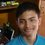# Powers Of Three, Oh Well!

Can anyone please help me solve this problem? I have been stuck with this for a long time and am unable to solve it.

The expression:

$3^{9} + 3^{12} + 3^{15} + 3^{n}$

is a perfect cube for some natural number $n$. Find the value of $n$.Note by Nilabha Saha
4 years, 8 months ago

This discussion board is a place to discuss our Daily Challenges and the math and science related to those challenges. Explanations are more than just a solution — they should explain the steps and thinking strategies that you used to obtain the solution. Comments should further the discussion of math and science.

When posting on Brilliant:

• Use the emojis to react to an explanation, whether you're congratulating a job well done , or just really confused .
• Ask specific questions about the challenge or the steps in somebody's explanation. Well-posed questions can add a lot to the discussion, but posting "I don't understand!" doesn't help anyone.
• Try to contribute something new to the discussion, whether it is an extension, generalization or other idea related to the challenge.

MarkdownAppears as
*italics* or _italics_ italics
**bold** or __bold__ bold
- bulleted- list
• bulleted
• list
1. numbered2. list
1. numbered
2. list
Note: you must add a full line of space before and after lists for them to show up correctly
paragraph 1paragraph 2

paragraph 1

paragraph 2

[example link](https://brilliant.org)example link
> This is a quote
This is a quote
    # I indented these lines
# 4 spaces, and now they show
# up as a code block.

print "hello world"
# I indented these lines
# 4 spaces, and now they show
# up as a code block.

print "hello world"
MathAppears as
Remember to wrap math in $$ ... $$ or $ ... $ to ensure proper formatting.
2 \times 3 $2 \times 3$
2^{34} $2^{34}$
a_{i-1} $a_{i-1}$
\frac{2}{3} $\frac{2}{3}$
\sqrt{2} $\sqrt{2}$
\sum_{i=1}^3 $\sum_{i=1}^3$
\sin \theta $\sin \theta$
\boxed{123} $\boxed{123}$

Sort by:

$3^{9} + 3^{12} + 3^{15} + 3^{n} = 3^9(1 + 3^3+3^6+3^{n-9})$.
$(a+b)^3=a^3 + 3 a^2 b + 3 a b^2 + b^3$.

$(3^k+1)^3 = 3^{3k} + 3^{2k+1}+3^{k+1}+1 = 1+ 3^3+3^6+3^{n-9}$
It works for $k=2, n=14$.

- 4 years, 8 months ago

Thank you very much. You literally releived me from an enthralling problem!

- 4 years, 8 months ago

Nice factorization! Are there other values of $n$ that would work?

I can show that $n = 3m$ would not work, but the other cases seem somewhat hairy.

Staff - 4 years, 8 months ago

I think it can be shown that the number to be cubed needs to be in a form $(9n+1)3^3$. Wolfram alpha shows just one solution. For a proper proof you might need some number theory experts on Brilliant.

- 4 years, 8 months ago

I'd start by thinking that that looks like a trinomial expansion. So let's take our cubed result and write it as 3^3 + a.

So we have 3^9 + 3^12 + 3^15 + 3^n = (3^3 + a)^3

=> 3^9 + 3^12 + 3^15+3^n = 3^9 + 3a3^6 + 3a^2 3^3 + a^3

=> 3^9 + 3^12 + 3^15+3^n = 3^9 + a3^7 + a^2 3^4 + a^3

Which fits if a = 3^5, and n = 14.

You can show that those are the only values by considering the graphs (of y = (fixed point + 3^x) and y=x^3) - they will only cross at one point.

- 4 years, 6 months ago

We can find n by successive substitution as follows(without thinking too much). Let 3^9 + 3^12 + 3^15 + 3^n = t^3. t must be divisible by 3, so let t =3r, and substitute: 3^9 + 3^12 + 3^15 + 3^n = (3r)^3 = 3^3*r^3. Dividing by 3^3, 3^6 + 3^9 + 3^12 +3^(n-3) = r^3. r must be divisible by 3, so let r = 3s, and substitute. Saving the reader a bit, s must be divisible by 3, so let s = 3u. We arrive at the following equation: 1 + 3^3 + 3^6 + 3^(n-9) = u^3. u must be of the form u = 3x + 1. Cubing, u^3 = 27x^3 + 27x^2 + 9x + 1, so 1 + 3^3 + 3^6 + 3^(n-9) = 27x^3 + 27x^2 + 9x + 1. Letting x = 3, 1 + 27 +729 + 3^(n-9) = 729 + 243 +27 + 1, or 3^(n-9) = 243 , or n-9 =5, n = 14. Ed Gray

- 3 years, 8 months ago

How do you know that's all of the possible values? IE Why can't there be another $x$ value that works?

Staff - 3 years, 8 months ago

3^9,3^12,3^15.....9+3=12+3=15+3=18...so,I hope the answer is 18....

- 4 years, 8 months ago

The answer is not 18. It is 14. The answer can't be found by assuming a geometric progression.

- 4 years, 8 months ago

yeap i understand

- 4 years, 8 months ago